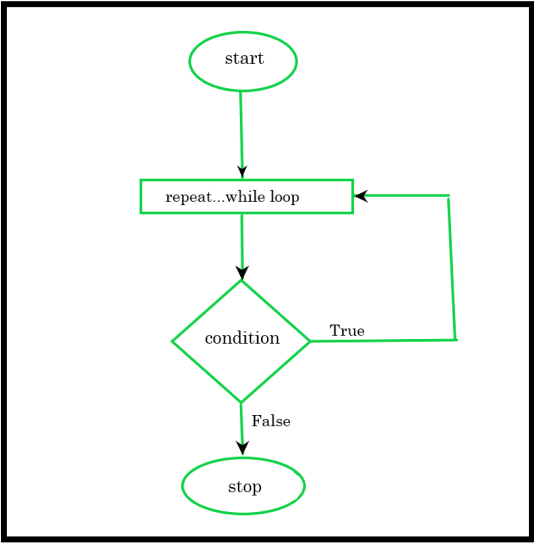# Swift – Repeat While loop

• Difficulty Level : Expert
• Last Updated : 09 Dec, 2021

Sometimes there’s a situation that comes when we have to execute a block of many numbers of times so to do this task we use the Repeat While loop. Or we can say that in Swift, we use the Repeat While loop to execute a block of code or a set of statements repeatedly. Repeat…while loop is almost same as while loop but with only one key difference i.e. the repeat-while loop will execute the statements at least one time even if the condition is FALSE because it will execute the statements first then it will check the condition but in while loop, statements execution will perform only when the condition is TRUE.

Syntax:

```repeat
{
// body of loop
// statements

} while (condition)```

Here,

• In Swift, the body of the loop or statement is executed first then the condition is checked.
• If the condition is true, the body of the loop inside the repeat statement is executed again.
• This process will continue until the condition evaluates to false.
• The loop will stop when the condition is FALSE.

Note: In Swift programming language the repeat-while loop is the same as the do…while loop in other programming languages.

Flowchart:Example 1:

## Swift

 `// Swift program to illustrate the use``// repeat...while loop``import` `Swift`` ` `// Creating variables``var` `number = 1, n = 10`` ` `// repeat...while loop from 1 to 10``print``(``"Numbers:"``)``repeat ``{`` ` `  ``// Statement``  ``print``(number)``  ``number = number + 1`` ` `}``while` `(number <= n)`

Output:

```Numbers:
1
2
3
4
5
6
7
8
9
10```

Explanation: In the above example, we have declared a variable number with value 1 and another variable n with value 10. The statement inside the repeat….while loop is executed first without checking the condition and then it will perform condition check and execute statements continuously till the condition is TRUE.

Example 2:

## Swift

 `// Swift program to illustrate the use``// repeat...while loop``import` `Swift`` ` `// Creating a variable``var` `number = 1`` ` `// Display GeeksforGeeks``// Using repeat while loop``repeat ``{`` ` `  ``// Statement``  ``print``(number, ``" GeeksForGeeks"``)``  ``number = number + 1`` ` `} ``while` `(number <= 5)`

Output:

```1 GeeksForGeeks
2 GeeksForGeeks
3 GeeksForGeeks
4 GeeksForGeeks
5 GeeksForGeeks```

Explanation: In the above example, we have declared a variable number with the value 1. In repeat while loop, first the statement is executed then the condition in checked and runs till the condition is true. In this example, the statement is executed first without checking any condition and prints (1 GeeksForGeeks ) and increases the value of the number by 2 ( number = number + 1 ). Now the value of the number is 2. Then first time condition is checked i.e. ( if 2 <= 5 ), the condition is TRUE, it will again move inside the repeat loop and this process will continue until the condition evaluates to false.  When the condition is FALSE it will terminate the loop.

My Personal Notes arrow_drop_up